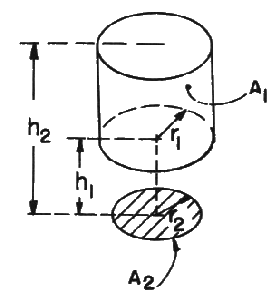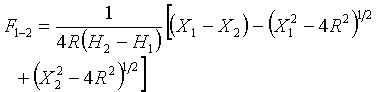# SECTION C Factors From Finite Areas to Finite Areas

## C-82: Interior surface of circular cylinder of radius R to disk of radius r where r < R; disk is perpendicular to axis of cylinder, and axis passes through center of disk.Definitions:

 R = r1/r2; H1 = h1/r2 H2 = h2/r2 X = H2 + R2 + 1

Governing Equation:To download a copy of this equation in MS Word 97 Format, click HERE.